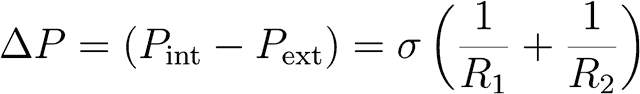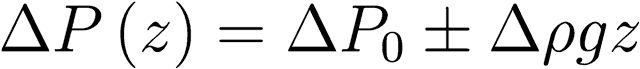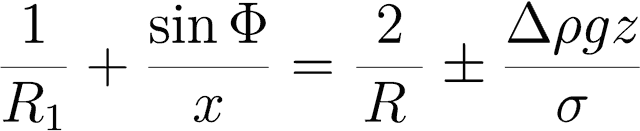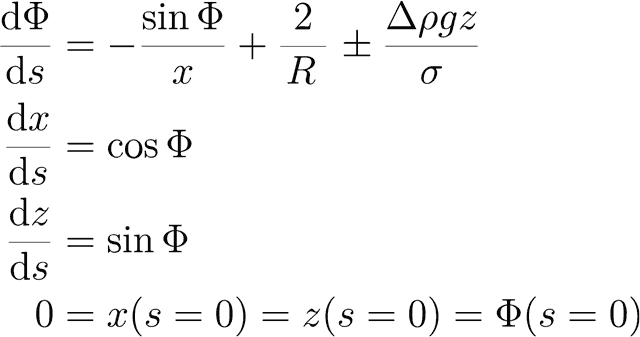Pendant drop method# Pendant drop method — Optical determination of the surface/interfacial tension

The surface tension of a liquid and the interfacial tension between two liquids can be determined with an optical contact angle measuring and contour analysis system of the OCA series. The setup drafted in figure 1 is used to capture an image of a liquid drop that hangs on a dosing needle and to subsequently analyse it with the DataPhysics Instruments software. The corresponding evaluation process is called pendant drop method.

The analysis of the drop shape is based on the Young-Laplace equation. This equation describes the pressure difference (Laplace pressure) between the areas inside and outside of a curved liquid surface/interface with the principal radii of curvature Ri:The forces that determine the shape of the pendant drop are in particular the surface tension and gravitation. The surface tension seeks to minimize the surface area and get the drop into a spherical shape. Gravitation on the other hand stretches the drop from this spherical shape and the typical pear-like shape results.

Gravitation causes a pressure difference across the z-axis according to Pascal's law (hydrostatic pressure). Therefore the Laplace pressure ΔP(z) at a distance z from an arbitrary reference plane with Laplace pressure ΔP0 is:Figure 1: Schematic setup for the pendant drop method

For a pendant drop the principal radii of curvature at the vertex (lowest point of the drop) are: R1=R2=R. Thus it is convenient to place the reference plane in this point. For every point above it holds R2=x/sin Φ (see figure 2). The mentioned equations lead to:The introduction of a parametrisation using the arc length s of the drop shape results in the following set of three first-order differential equations with three boundary values, which is solvable by numerical procedures:The numerical fit of the theoretical drop shape to the shape recorded by the camera eventually yields the surface tension. The described considerations also apply when the surrounding phase is not air but another liquid. In this case the interfacial tension is evaluated, respectively.

The Young-Laplace fit can also be used to analyse the deformation of a drop sitting on a solid surface for determining the contact angle in the sessile drop method.

Figure 2: For the derivation of the Young-Laplace fit on a pendant drop

# Chapters

Using the following buttons you can navigate between the individual chapters of our knowledge-base Understanding Interfaces